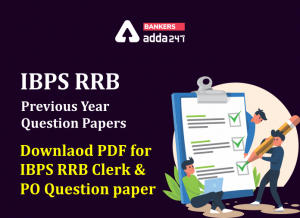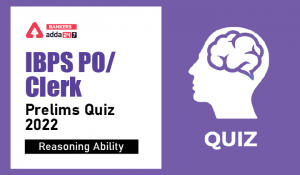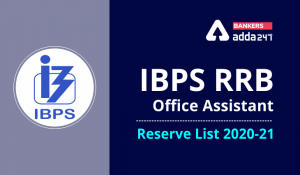Latest Banking jobs   »   Reasoning Ability Quiz For IBPS RRB...

# Reasoning Ability Quiz For IBPS RRB PO, Clerk Prelims 2021- 17th May

Directions (1-5): In this question, relationship between different elements is shown in the statements. The statements are followed by conclusions. Study the conclusions based on the given statement and select the appropriate answer.

Q1. Statements: P>Q>R=U, U≥T<S, T≥W
Conclusions:
I. R>W
II. U=W
(a) Only conclusion I is true
(b) Only conclusion II is true
(c) Either conclusion I or II is true
(d) Both conclusions I and II are true
(e) Neither conclusion I nor II is true

Q2. Statements: P>Q>R<U, U≥V>S, V<W
Conclusions:
I. P>S
II. P < S
(a) Only conclusion I is true
(b) Only conclusion II is true
(c) Either conclusion I or II is true
(d) Both conclusions I and II are true
(e) Neither conclusion I nor II is true

Q3. Statements: A = K > X < L > N ≤ R; Q ≥ A; N = M
Conclusions:
I. Q > X
II. K > M
(a) Only conclusion I is true
(b) Only conclusion II is true
(c) Either conclusion I or II is true
(d) Both conclusions I and II are true
(e) Neither conclusion I nor II is true

Q4. Statements: J = K > X < L > N ≤ R; Q ≥ K; J < M
Conclusions:
I. Q > X
II. X < M
(a) Only conclusion I is true
(b) Only conclusion II is true
(c) Either conclusion I or II is true
(d) Both conclusions I and II are true
(e) Neither conclusion I nor II is true

Q5. Statements: J >K > X < L > N ≤ R; Q ≥ J < M
Conclusions:
I. R < M
II. M ≥ R
(a) Only conclusion I is true
(b) Only conclusion II is true
(c) Either conclusion I or II is true
(d) Both conclusions I and II are true
(e) Neither conclusion I nor II is true

Directions (6-8): In the given questions, assuming the given statements to be true. Find which of the given two conclusions numbered I, II is/are definitely true and give your answer accordingly.

Q6. Statements: H = W≤ R, R > F
Conclusions: I. R = H
II. R > H
(a) Both I and II are true
(b) Only II is true
(c) Only I is true.
(d) Either I or II is true.
(e) None is true.

Q7. Statements: Z<R ≤N, N ≥F, F > B
Conclusions: I. F = R
II. B < N
(a) Both I and II are true
(b) Only I is true
(c) Only II is true
(d) Either I or II is true.
(e) None is true.

Q8. Statements: S>H > W, W < M, M ≥K
Conclusions: I. K < W
II.H > M
(a) None is true
(b) Only II is true
(c) Only I is true.
(d) Either I or II is true.
(e) Both I and II are true

Direction (9-10): In these questions, relationship between different elements is show in the statements. The statements are followed by conclusions. Study the conclusions based on the given statements and select the appropriate answer:
(a) If only conclusion I follows.
(b) If only conclusion II follows.
(c) If either conclusion I or II follows
(d) If neither conclusion I nor II follows.
(e) If both conclusions I and II follow.

Q9. Statements: W>E=G>B<C<L≤K<J=S
Conclusion I: B≤K II: W>B

Q10. Statements: Q>S>D>C>X=M>U<K=L
Conclusion I: Q>M II: K>X

Directions (11-15): In these questions, relationship between different elements is shown in the statements. These statements are followed by two conclusions.
(a) If only conclusion I follows.
(b) If only conclusion II follows.
(c) If either conclusion I or II follows.
(d) If neither conclusion I nor II follows.
(e) If both conclusions I and II follow.

Q11. Statements: A ≤ E, F ≥ B < C < A
Conclusions: I. B < E II. F > A

Q12. Statements: G > O, K ≥ G < F ≤ L < M
Conclusions: I. O ≥ L II. K ≥ O

Q13. Statements: V > Q> M > X= S, Q ≥ T >N
Conclusions: I. N > X II. N ≤ S

Q14. Statements: R ≥ Y > Q, U < Q, X < Y ≥ P
Conclusions: I. X > U II. P ≤ R

Q15. Statements: O ≥ J < Y = Z > I > R
Conclusions: I. O ≥ I II. R < Y

Practice More Questions of Reasoning for Competitive Exams:

###### Study Plan for IBPS RRB PO/Clerk Prelims 2021

Solutions

S1. Ans.(c)
Sol. I. R > W (False) II. U = W (False)

S2. Ans.(e)
Sol. I. P > S (False) II. P < S (False)

S3. Ans.(a)
Sol. I. Q > X(True) II. K > M (False)

S4. Ans.(d)
Sol. I. Q > X (True) II. X < M (True)

S5. Ans.(e)
Sol. I. R < M(False) II. M ≥ R (False)

S6. Ans. (d)
Sol. I. R = H (False) II. R > H (False)

S7. Ans. (c)
Sol. I. F = R (False) II. B < N (True)

S8. Ans. (a)
Sol. I. K < W (False) II. H > M (False)

S9.Ans(b)
Sol. I: B≤K(False) II: W>B(True)

S10.Ans(a)
Sol. I: Q>M(True) II: K>X(False)

S11. Ans.(a)
Sol. I. B < E(true) II. F > A(false)

S12. Ans.(d)
Sol. I. O ≥ L(false) II. K ≥ O(false)

S13. Ans.(c)
Sol. I. N > X(false) II. N ≤ S(false)

S14. Ans.(b)
Sol. I. X > U(false) II. P ≤ R(true)

S15. Ans.(b)
Sol. I. O ≥ I(false) II. R < Y(true)#### Congratulations!Incorrect details? Fill the form again here

•IBPS RRB Previous Year Question Paper PD...
•Reasoning Ability Quiz For IBPS Clerk/PO...
•Reasoning Ability Quiz For IBPS RRB PO C...
•Reasoning Ability Quiz For IBPS Clerk Pr...
•Reasoning Ability Quiz For IBPS RRB PO C...
•IBPS RRB Clerk 2nd Reserve List 2022 Out...